### Home > PC > Chapter 6 > Lesson 6.1.2 > Problem6-32

6-32.
1. Copy and replace the question mark with the correct number, or state “impossible” and explain why. Homework Help ✎

1. log2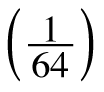= ?

2. log8(?) = 0

3. log8(8) = ?

4. log2(64) = ?

5. log8(−8) = ?

6. log5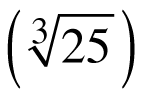= ?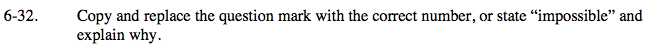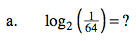Rewrite the expression in exponential form.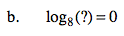Change the problem to exponential form.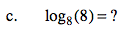Change the problem to exponential form.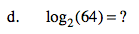Write the argument in exponential form.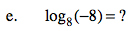What is the domain of a log function?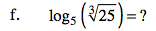Write the argument in exponential form.